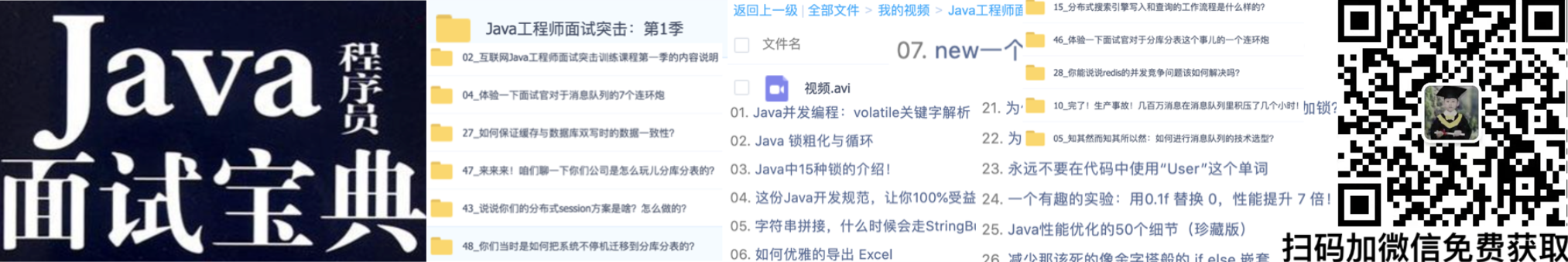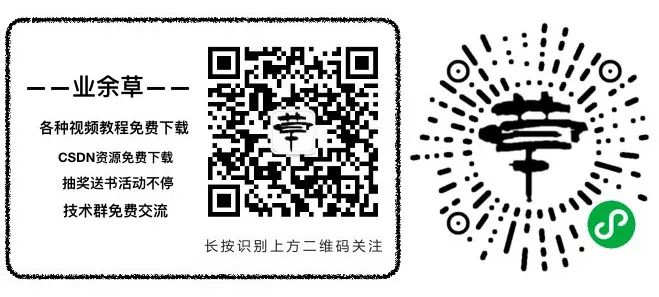# 排序算法之鸡尾酒排序

JAVA 1698浏览• 先对数组从左到右进行冒泡排序（升序），则最大的元素去到最右端
• 再对数组从右到左进行冒泡排序（降序），则最小的元素去到最左端
• 以此类推，依次改变冒泡的方向，并不断缩小未排序元素的范围，直到最后一个元素结束

``````public static void cocktail_sort(int[] arr) {
int i, left = 0, right = arr.length - 1;
int temp;
while (left < right) {
for (i = left; i < right; i++)
if (arr[i] > arr[i + 1]) {
temp = arr[i];
arr[i] = arr[i + 1];
arr[i + 1] = temp;
}
right--;
for (i = right; i > left; i--)
if (arr[i - 1] > arr[i]) {
temp = arr[i];
arr[i] = arr[i - 1];
arr[i - 1] = temp;
}
left++;
}
}``````